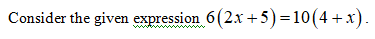# 6(2x + 5)  = 10(4 + x)  then  x =Select one:a. 15b. 5c. 20d. 10

Question
1 views

6(2x + 5)  = 10(4 + x)  then  x =

Select one:
a. 15
b. 5
c. 20
d. 10
check_circle

Step 1Step 2

The value of x is evaluate...

### Want to see the full answer?

See Solution

#### Want to see this answer and more?

Solutions are written by subject experts who are available 24/7. Questions are typically answered within 1 hour.*

See Solution
*Response times may vary by subject and question.
Tagged in

### Math